Updating search results...

# 33 Results

View
Selected filters:
• HS MathUnrestricted Use
CC BY
Rating
0,0 stars

This task examines the ways in which the plane can be covered by regular polygons in a very strict arrangement called a regular tessellation. These tessellations are studied here using algebra, which enters the picture via the formula for the measure of the interior angles of a regular polygon (which should therefore be introduced or reviewed before beginning the task). The goal of the task is to use algebra in order to understand which tessellations of the plane with regular polygons are possible.

نوع المادة:
Activity/Lab
Provider:
Illustrative Mathematics
Provider Set:
Illustrative Mathematics
المؤلف:
Illustrative Mathematics
01/21/2013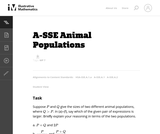Unrestricted Use
CC BY
Rating
0,0 stars

In this task students have to interpret expressions involving two variables in the context of a real world situation. All given expressions can be interpreted as quantities that one might study when looking at two animal populations.

نوع المادة:
Activity/Lab
Provider:
Illustrative Mathematics
Provider Set:
Illustrative Mathematics
المؤلف:
Illustrative Mathematics
05/01/2012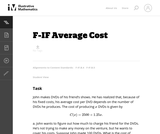Unrestricted Use
CC BY
Rating
0,0 stars

In this real world problem students solve questions based on the relationship between production costs and price.

نوع المادة:
Activity/Lab
Provider:
Illustrative Mathematics
Provider Set:
Illustrative Mathematics
المؤلف:
Illustrative Mathematics
05/01/2012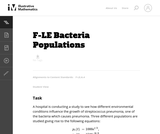Unrestricted Use
CC BY
Rating
0,0 stars

This task provides a real world context for interpreting and solving exponential equations. There are two solutions provided for part (a). The first solution demonstrates how to deduce the conclusion by thinking in terms of the functions and their rates of change. The second approach illustrates a rigorous algebraic demonstration that the two populations can never be equal.

نوع المادة:
Activity/Lab
Provider:
Illustrative Mathematics
Provider Set:
Illustrative Mathematics
المؤلف:
Illustrative Mathematics
05/01/2012Unrestricted Use
CC BY
Rating
0,0 stars

نوع المادة:
Activity/Lab
Provider:
Illustrative Mathematics
Provider Set:
Illustrative Mathematics
المؤلف:
Illustrative Mathematics
05/01/2012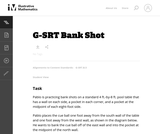Unrestricted Use
CC BY
Rating
0,0 stars

This task asks students to use similarity to solve a problem in a context that will be familiar to many, though most students are accustomed to using intuition rather than geometric reasoning to set up the shot.

نوع المادة:
Activity/Lab
Provider:
Illustrative Mathematics
Provider Set:
Illustrative Mathematics
المؤلف:
Illustrative Mathematics
08/21/2012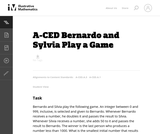Unrestricted Use
CC BY
Rating
0,0 stars

This task presents a simple but mathematically interesting game whose solution is a challenging exercise in creating and reasoning with algebraic inequalities. The core of the task involves converting a verbal statement into a mathematical inequality in a context in which the inequality is not obviously presented, and then repeatedly using the inequality to deduce information about the structure of the game.

نوع المادة:
Activity/Lab
Provider:
Illustrative Mathematics
Provider Set:
Illustrative Mathematics
المؤلف:
Illustrative Mathematics
10/21/2013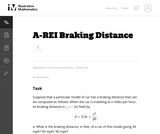Unrestricted Use
CC BY
Rating
0,0 stars

This task provides an exploration of a quadratic equation by descriptive, numerical, graphical, and algebraic techniques. Based on its real-world applicability, teachers could use the task as a way to introduce and motivate algebraic techniques like completing the square, en route to a derivation of the quadratic formula.

نوع المادة:
Activity/Lab
Provider:
Illustrative Mathematics
Provider Set:
Illustrative Mathematics
المؤلف:
Illustrative Mathematics
05/01/2012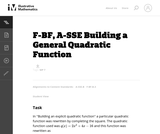Unrestricted Use
CC BY
Rating
0,0 stars

This task is for instructional purposes only and builds on ``Building an explicit quadratic function.''

نوع المادة:
Activity/Lab
Provider:
Illustrative Mathematics
Provider Set:
Illustrative Mathematics
المؤلف:
Illustrative Mathematics
08/20/2012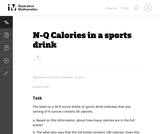Unrestricted Use
CC BY
Rating
0.0 stars

This problem involves the meaning of numbers found on labels. When the level of accuracy is not given we need to make assumptions based on how the information is reported. The goal of the task is to stimulate a conversation about rounding and about how to record numbers with an appropriate level of accuracy, tying in directly to the standard N-Q.3. It is therefore better suited for instruction than for assessment purposes.

Material Type:
Activity/Lab
Provider:
Illustrative Mathematics
Provider Set:
Illustrative Mathematics
Author:
Illustrative Mathematics
01/05/2013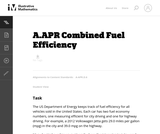Unrestricted Use
CC BY
Rating
0,0 stars

The primary purpose of this problem is to rewrite simple rational expressions in different forms to exhibit different aspects of the expression, in the context of a relevant real-world context (the fuel efficiency of of a car). Indeed, the given form of the combined fuel economy computation is useful for direct calculation, but if asked for an approximation, is not particularly helpful.

نوع المادة:
Activity/Lab
Provider:
Illustrative Mathematics
Provider Set:
Illustrative Mathematics
المؤلف:
Illustrative Mathematics
01/25/2013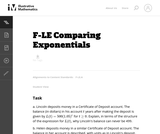Unrestricted Use
CC BY
Rating
0,0 stars

This task gives students an opportunity to work with exponential functions in a real world context involving continuously compounded interest. They will study how the base of the exponential function impacts its growth rate and use logarithms to solve exponential equations.

نوع المادة:
Activity/Lab
Provider:
Illustrative Mathematics
Provider Set:
Illustrative Mathematics
المؤلف:
Illustrative Mathematics
05/01/2012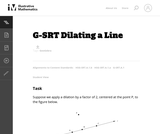Unrestricted Use
CC BY
Rating
0,0 stars

This task gives students the opportunity to verify that a dilation takes a line that does not pass through the center to a line parallel to the original line, and to verify that a dilation of a line segment (whether it passes through the center or not) is longer or shorter by the scale factor.

نوع المادة:
Activity/Lab
Provider:
Illustrative Mathematics
Provider Set:
Illustrative Mathematics
المؤلف:
Illustrative Mathematics
05/01/2012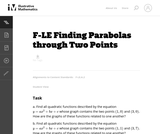Unrestricted Use
CC BY
Rating
0,0 stars

In this task students have the opportunity to construct linear and exponential functions, including arithmetic and geometric sequences, given a graph, a description of a relationship, or two input-output pairs (include reading these from a table).

نوع المادة:
Activity/Lab
Provider:
Illustrative Mathematics
Provider Set:
Illustrative Mathematics
المؤلف:
Illustrative Mathematics
05/01/2012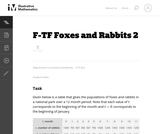Unrestricted Use
CC BY
Rating
0,0 stars

The example of rabbits and foxes was introduced in the task (8-F Foxes and Rabbits) to illustrate two functions of time given in a table. We are now in a position to actually model the data given previously with trigonometric functions and investigate the behavior of this predator-prey situation.

نوع المادة:
Activity/Lab
Provider:
Illustrative Mathematics
Provider Set:
Illustrative Mathematics
المؤلف:
Illustrative Mathematics
08/29/2012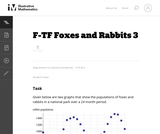Unrestricted Use
CC BY
Rating
0,0 stars

The example of rabbits and foxes was introduced in 8-F Foxes and Rabbits to illustrate two functions of time given in a table. The same situation was used in F-TF Foxes and Rabbits 2 to find trigonometric functions modeling the data in the table. The previous situation was somewhat unrealistic since we were able to find functions that fit the data perfectly. In this task, on the other hand, we do some legitimate modelling, in that we come up with functions that approximate the data well, but do not perfectly match, the given data.

نوع المادة:
Activity/Lab
Provider:
Illustrative Mathematics
Provider Set:
Illustrative Mathematics
المؤلف:
Illustrative Mathematics
08/30/2012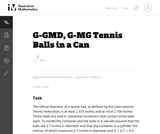Unrestricted Use
CC BY
Rating
0,0 stars

This task is inspired by the derivation of the volume formula for the sphere. If a sphere of radius 1 is enclosed in a cylinder of radius 1 and height 2, then the volume not occupied by the sphere is equal to the volume of a Ňdouble-naped coneÓ with vertex at the center of the sphere and bases equal to the bases of the cylinder.

نوع المادة:
Activity/Lab
Provider:
Illustrative Mathematics
Provider Set:
Illustrative Mathematics
المؤلف:
Illustrative Mathematics
05/01/2012Unrestricted Use
CC BY
Rating
0,0 stars

The purpose of this task is for students to apply the concepts of mass, volume, and density in a real-world context. There are several ways one might approach the problem, e.g., by estimating the volume of a person and dividing by the volume of a cell.

نوع المادة:
Activity/Lab
Provider:
Illustrative Mathematics
Provider Set:
Illustrative Mathematics
المؤلف:
Illustrative Mathematics
11/13/2012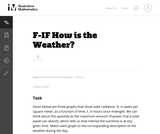Unrestricted Use
CC BY
Rating
0,0 stars

This task can be used as a quick assessment to see if students can make sense of a graph in the context of a real world situation. Students also have to pay attention to the scale on the vertical axis to find the correct match.

نوع المادة:
Activity/Lab
Provider:
Illustrative Mathematics
Provider Set:
Illustrative Mathematics
المؤلف:
Illustrative Mathematics
05/01/2012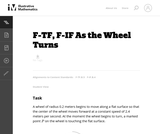Unrestricted Use
CC BY
Rating
0,0 stars

In this task, students use trigonometric functions to model the movement of a point around a wheel and, in the case of part (c), through space (F-TF.5). Students also interpret features of graphs in terms of the given real-world context (F-IF.4).

نوع المادة:
Activity/Lab
Provider:
Illustrative Mathematics
Provider Set:
Illustrative Mathematics
المؤلف:
Illustrative Mathematics
05/01/2012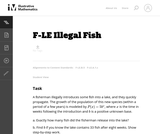Unrestricted Use
CC BY
Rating
0.0 stars

This is a direct task suitable for the early stages of learning about exponential functions. Students interpret the relevant parameters in terms of the real-world context and describe exponential growth.

Material Type:
Activity/Lab
Provider:
Illustrative Mathematics
Provider Set:
Illustrative Mathematics
Author:
Illustrative Mathematics
05/01/2012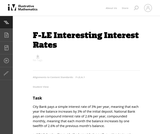Unrestricted Use
CC BY
Rating
0.0 stars

This real world task requires students to compare differing interest rates.

Material Type:
Activity/Lab
Provider:
Illustrative Mathematics
Provider Set:
Illustrative Mathematics
Author:
Illustrative Mathematics
05/01/2012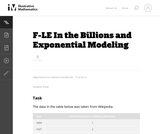Unrestricted Use
CC BY
Rating
0.0 stars

This problem provides an opportunity to experiment with modeling real data. Populations are often modeled with exponential functions and in this particular case we see that, over the last 200 years, the rate of population growth accelerated rapidly, reaching a peak a little after the middle of the 20th century and now it is slowing down.

Material Type:
Activity/Lab
Provider:
Illustrative Mathematics
Provider Set:
Illustrative Mathematics
Author:
Illustrative Mathematics
05/01/2012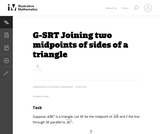Unrestricted Use
CC BY
Rating
0.0 stars

This task is closely related to very important material about similarity and ratios in geometry.

Material Type:
Activity/Lab
Provider:
Illustrative Mathematics
Provider Set:
Illustrative Mathematics
Author:
Illustrative Mathematics
01/21/2013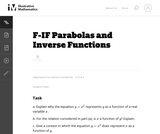Unrestricted Use
CC BY
Rating
0.0 stars

This task assumes students have an understanding of the relationship between functions and equations. Using this knowledge, the students are prompted to try to solve equations in order to find the inverse of a function given in equation form: when no such solution is possible, this means that the function does not have an inverse.

Material Type:
Activity/Lab
Provider:
Illustrative Mathematics
Provider Set:
Illustrative Mathematics
Author:
Illustrative Mathematics
06/08/2012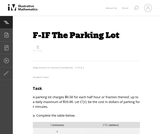Unrestricted Use
CC BY
Rating
0.0 stars

The purpose of this task is to investigate the meaning of the definition of function in a real-world context where the question of whether there is more than one output for a given input arises naturally. In more advanced courses this task could be used to investigate the question of whether a function has an inverse.

Material Type:
Activity/Lab
Provider:
Illustrative Mathematics
Provider Set:
Illustrative Mathematics
Author:
Illustrative Mathematics
05/01/2012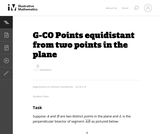Unrestricted Use
CC BY
Rating
0.0 stars

This task is part of a series presenting important foundational geometric results and constructions which are fundamental for more elaborate arguments. They are presented without a real world context so as to see the important hypotheses and logical steps involved as clearly as possible.

Material Type:
Activity/Lab
Provider:
Illustrative Mathematics
Provider Set:
Illustrative Mathematics
Author:
Illustrative Mathematics
01/11/2013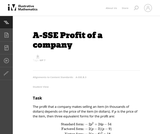Unrestricted Use
CC BY
Rating
0.0 stars

This task compares the usefulness of different forms of a quadratic expression. Students have to choose which form most easily provides information about the maximum value, the zeros and the vertical intercept of a quadratic expression in the context of a real world situation. Rather than just manipulating one form into the other, students can make sense out of the structure of the expressions.

Material Type:
Activity/Lab
Provider:
Illustrative Mathematics
Provider Set:
Illustrative Mathematics
Author:
Illustrative Mathematics
05/01/2012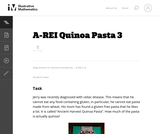Unrestricted Use
CC BY
Rating
0.0 stars

This tasks is an example of a mathematical modeling problem (SMP 4) and it also illustrates SMP 1 (Making sense of a problem). Students are only told that there are two ingredients in the pasta and they have a picture of the box. It might even be better to just show the picture of the box, or to bring in the box and ask the students to pose the question themselves.

Material Type:
Activity/Lab
Provider:
Illustrative Mathematics
Provider Set:
Illustrative Mathematics
Author:
Illustrative Mathematics
09/13/2012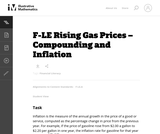Unrestricted Use
CC BY
Rating
0.0 stars

The purpose of this task is to give students an opportunity to explore various aspects of exponential models (e.g., distinguishing between constant absolute growth and constant relative growth, solving equations using logarithms, applying compound interest formulas) in the context of a real world problem with ties to developing financial literacy skills.

Material Type:
Activity/Lab
Provider:
Illustrative Mathematics
Provider Set:
Illustrative Mathematics
Author:
Illustrative Mathematics
02/13/2013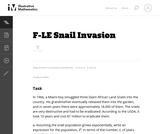Unrestricted Use
CC BY
Rating
0.0 stars

The purpose of this task is to give students experience modeling a real-world example of exponential growth, in a context that provides a vivid illustration of the power of exponential growth, for example the cost of inaction for a year.

Material Type:
Activity/Lab
Provider:
Illustrative Mathematics
Provider Set:
Illustrative Mathematics
Author:
Illustrative Mathematics
05/01/2012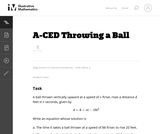Unrestricted Use
CC BY
Rating
0.0 stars

Although this task is quite straightforward, it has a couple of aspects designed to encourage students to attend to the structure of the equation and the meaning of the variables in it. It fosters flexibility in seeing the same equation in two different ways, and it requires students to attend to the meaning of the variables in the preamble and extract the values from the descriptions.

Material Type:
Activity/Lab
Provider:
Illustrative Mathematics
Provider Set:
Illustrative Mathematics
Author:
Illustrative Mathematics
05/01/2012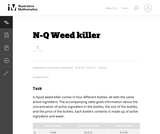Unrestricted Use
CC BY
Rating
0.0 stars

The principal purpose of the task is to explore a real-world application problem with algebra, working with units and maintaining reasonable levels of accuracy throughout.

Material Type:
Activity/Lab
Provider:
Illustrative Mathematics
Provider Set:
Illustrative Mathematics
Author:
Illustrative Mathematics